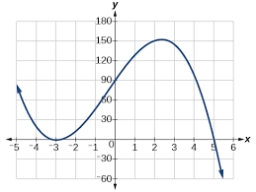# Consumption 58231

Assume that consumption C depends on income y according to the function C = a + by, where a and b are parameters. The slope of this function is 0.8, and c is €50 when Y is €30. Does it apply to the values ​​of parameters a and b?

b =  0.8
a =  26

### Step-by-step explanation:Did you find an error or inaccuracy? Feel free to write us. Thank you!

Tips for related online calculators
The line slope calculator is helpful for basic calculations in analytic geometry. The coordinates of two points in the plane calculate slope, normal and parametric line equation(s), slope, directional angle, direction vector, the length of the segment, intersections of the coordinate axes, etc.